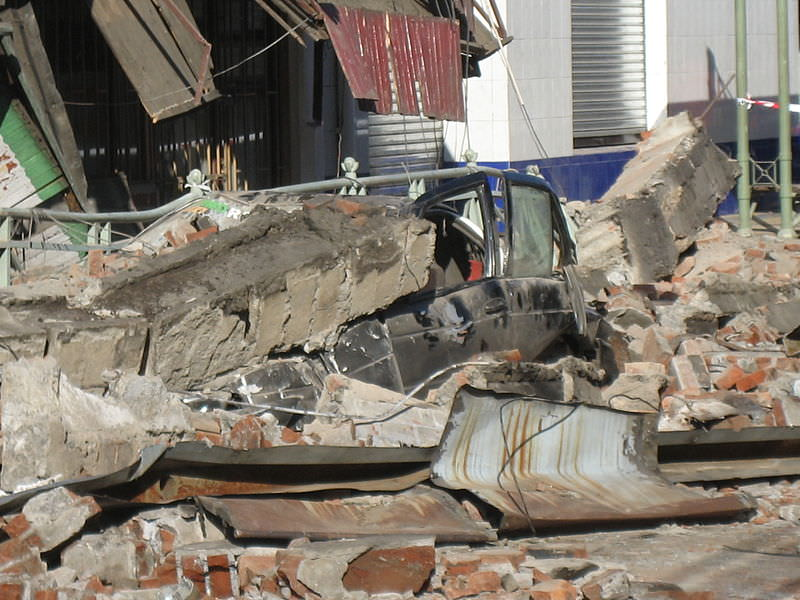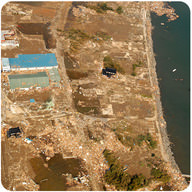# 6.18: Earthquake Magnitude Scales

•• Contributed by CK12
• CK12How do scientists measure earthquakes?

This 8.8 magnitude earthquake in Chile in 2010 caused over 500 deaths and thousands of injuries. Earthquakes and the damage they cause can be measured in a few different ways. One way is to describe the damage. The other way is to measure the energy of the quake.

## Earthquake Intensity

The ways seismologists measure an earthquake have changed over the decades. Initially, they could measure only what people felt and saw: the intensity. For this, they used the Mercalli scale.

### Mercalli Scale

Early in the 20th century, earthquakes were described in terms of what people felt and the damage that was done to buildings. The Mercalli intensity scale describes earthquake intensity.

There are many problems with the Mercalli scale. The damage from an earthquake is affected by many things. The type of ground a building sits on is very important to what happens to that building in a quake. Different people experience an earthquake differently. Using this scale, comparisons between earthquakes were difficult to make. A new scale was needed.

## Earthquake Magnitude

For decades scientists have had equipment that can measure earthquake magnitude. The earthquake magnitude is the energy released during the quake.

### The Richter Magnitude Scale

Charles Richter developed the Richter magnitude scale in 1935. The Richter scale measures the magnitude of an earthquake's largest jolt of energy. This is determined by using the height of the waves recorded on a seismograph.

The Richter scale is logarithmic. The magnitudes jump from one level to the next. The height of the largest wave increases 10 times with each level. So the height of the largest seismic wave of a magnitude 5 quake is 10 times that of a magnitude 4 quake. A magnitude 5 is 100 times that of a magnitude 3 quake. With each level, thirty times more energy is released. A difference of two levels on the Richter scale equals 900 times more released energy.

The Richter scale has limitations. A single sharp jolt measures higher on the Richter scale than a very long intense earthquake. Yet, this is misleading because the longer quake releases more energy. Earthquakes that release more energy are likely to do more damage. As a result, another scale was needed.

### The Moment Magnitude Scale

The moment magnitude scale is the favored method of measuring earthquake magnitudes. It measures the total energy released by an earthquake. Moment magnitude is calculated by two things. One is the length of the fault break. The other is the distance the ground moves along the fault. Japan's Tōhoku earthquake in 2011 had a magnitude of 9.0 (Figure below).Earthquake and tsunami damage in Japan, 2011. The Tōhoku earthquake had a magnitude of 9.0.

## Summary

• Mercalli Intensity Scale depends on many factors other than the amount of energy released in the earthquake. These factors include the type of rock in the region. They also include the quality of the structures built in the area.
• The Richter scale is a logarithmic scale that measures the largest jolt of energy released by an earthquake.
• The moment magnitude scale is a logarithmic scale that measures the total amount of energy released by an earthquake.

## Review

1. Under what circumstances might the Mercalli intensity scale be useful today? Why was it replaced by the Richter and then the moment magnitude scales?
2. Why do scientists prefer the moment magnitude scale to the Richter scale?
3. In 2011 there was a 5.8 magnitude quake that struck Virginia and a 9.0 quake that struck Japan. How might a 5.8 quake rate on the Mercalli scale in Virginia? How might a 5.8 magnitude quake rate on the Mercalli scale in Japan?

## Explore More

Use the resource below to answer the questions that follow.

1. What was the magnitude of the 2010 Haiti earthquake?
2. What does magnitude mean?
3. How much larger is each earthquake magnitude?
4. What is the difference between two magnitudes?
5. What was the largest earthquake ever measured?